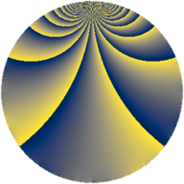# Properties

 Label 546.2.qLevel $546$ Weight $2$ Character orbit 546.q Rep. character $\chi_{546}(251,\cdot)$ Character field $\Q(\zeta_{6})$ Dimension $72$ Newform subspaces $10$ Sturm bound $224$ Trace bound $3$

# Related objects

## Defining parameters

 Level: $$N$$ $$=$$ $$546 = 2 \cdot 3 \cdot 7 \cdot 13$$ Weight: $$k$$ $$=$$ $$2$$ Character orbit: $$[\chi]$$ $$=$$ 546.q (of order $$6$$ and degree $$2$$) Character conductor: $$\operatorname{cond}(\chi)$$ $$=$$ $$273$$ Character field: $$\Q(\zeta_{6})$$ Newform subspaces: $$10$$ Sturm bound: $$224$$ Trace bound: $$3$$ Distinguishing $$T_p$$: $$5$$, $$11$$, $$17$$

## Dimensions

The following table gives the dimensions of various subspaces of $$M_{2}(546, [\chi])$$.

Total New Old
Modular forms 240 72 168
Cusp forms 208 72 136
Eisenstein series 32 0 32

## Trace form

 $$72 q - 36 q^{4} - 4 q^{9} + O(q^{10})$$ $$72 q - 36 q^{4} - 4 q^{9} - 12 q^{15} - 36 q^{16} - 56 q^{25} - 16 q^{30} - 4 q^{36} + 48 q^{39} + 16 q^{42} - 20 q^{43} + 12 q^{46} - 12 q^{49} - 48 q^{51} + 96 q^{63} + 72 q^{64} - 84 q^{67} + 12 q^{72} - 44 q^{78} + 16 q^{79} + 56 q^{81} - 120 q^{85} + 32 q^{91} + 48 q^{93} + O(q^{100})$$

## Decomposition of $$S_{2}^{\mathrm{new}}(546, [\chi])$$ into newform subspaces

Label Dim $A$ Field CM Traces $q$-expansion
$a_{2}$ $a_{3}$ $a_{5}$ $a_{7}$
546.2.q.a $2$ $4.360$ $$\Q(\sqrt{-3})$$ None $$-1$$ $$-3$$ $$0$$ $$1$$ $$q+(-1+\zeta_{6})q^{2}+(-1-\zeta_{6})q^{3}-\zeta_{6}q^{4}+\cdots$$
546.2.q.b $2$ $4.360$ $$\Q(\sqrt{-3})$$ None $$-1$$ $$3$$ $$0$$ $$5$$ $$q+(-1+\zeta_{6})q^{2}+(1+\zeta_{6})q^{3}-\zeta_{6}q^{4}+\cdots$$
546.2.q.c $2$ $4.360$ $$\Q(\sqrt{-3})$$ None $$1$$ $$-3$$ $$0$$ $$1$$ $$q+(1-\zeta_{6})q^{2}+(-1-\zeta_{6})q^{3}-\zeta_{6}q^{4}+\cdots$$
546.2.q.d $2$ $4.360$ $$\Q(\sqrt{-3})$$ None $$1$$ $$3$$ $$0$$ $$5$$ $$q+(1-\zeta_{6})q^{2}+(1+\zeta_{6})q^{3}-\zeta_{6}q^{4}+\cdots$$
546.2.q.e $4$ $4.360$ $$\Q(\sqrt{-3}, \sqrt{-11})$$ None $$-2$$ $$-1$$ $$0$$ $$2$$ $$q-\beta _{2}q^{2}+(-1+\beta _{1}+\beta _{2})q^{3}+(-1+\cdots)q^{4}+\cdots$$
546.2.q.f $4$ $4.360$ $$\Q(\sqrt{-3}, \sqrt{-11})$$ None $$-2$$ $$1$$ $$0$$ $$10$$ $$q+(-1+\beta _{2})q^{2}+(\beta _{2}+\beta _{3})q^{3}-\beta _{2}q^{4}+\cdots$$
546.2.q.g $4$ $4.360$ $$\Q(\sqrt{-3}, \sqrt{-11})$$ None $$2$$ $$-2$$ $$0$$ $$2$$ $$q+\beta _{2}q^{2}+(-\beta _{1}+\beta _{3})q^{3}+(-1+\beta _{2}+\cdots)q^{4}+\cdots$$
546.2.q.h $4$ $4.360$ $$\Q(\sqrt{-3}, \sqrt{-11})$$ None $$2$$ $$2$$ $$0$$ $$10$$ $$q+\beta _{2}q^{2}+(1-\beta _{1}+\beta _{3})q^{3}+(-1+\beta _{2}+\cdots)q^{4}+\cdots$$
546.2.q.i $24$ $4.360$ None $$-12$$ $$0$$ $$0$$ $$-18$$
546.2.q.j $24$ $4.360$ None $$12$$ $$0$$ $$0$$ $$-18$$

## Decomposition of $$S_{2}^{\mathrm{old}}(546, [\chi])$$ into lower level spaces

$$S_{2}^{\mathrm{old}}(546, [\chi]) \cong$$ $$S_{2}^{\mathrm{new}}(273, [\chi])$$$$^{\oplus 2}$$